# J.J. Thomson Experiment To Determine The Specific Charge Of An Electron (Cathode Ray)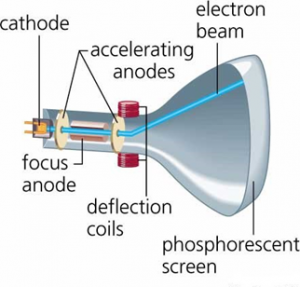The specific charge of an electron can be determined when electron moves in both magnetic field and electric field which are mutually perpendicular to each other so that the net force on the electron is made zero. IN this situation the direction of motion of electron remains perpendicular to both electric and magnetic field.

Construction:
The experimental setup shown in the figure shows the modern J.J. Thomson experiment to determine the specific charge of the electron. It consists of discharge tube in which two electrodes anode (A) and cathode(C) are present P1 and P2 are two parallel electric plates to generate electric field strength (E).
Working Principle: (Theory):
When sufficient amount of potential (V) is applied between the two electrodes electrons emitted from the cathode accelerate with velocity ‘v’ then,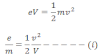When the charge particle moves under the action of electric field strength (E) it moves towards the +ve plate and finally incident at point O, at fluorescent screen. In case of applying magnetic field only, the charge particle deviate and finally incident at 02 as shown in figure.
If both magnetic and electric field are applied and their magnitude and direction is adjusted so that the charged particles move without deviation. At this condition charge particle incident at point O at the fluorescent screen,
Then,
We have,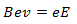Where, B is magnetic field strength. In this case the direction of magnetic field is perpendicular to the direction of the motion of the charged particle.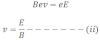From equation (i) and (ii),
We get,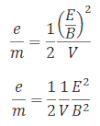Hence, the specific charge at the electron is determined if the values of E, B and V are known.
The experimentally obtained specific charge of the electron is 1.7 into 1011 c/kg.